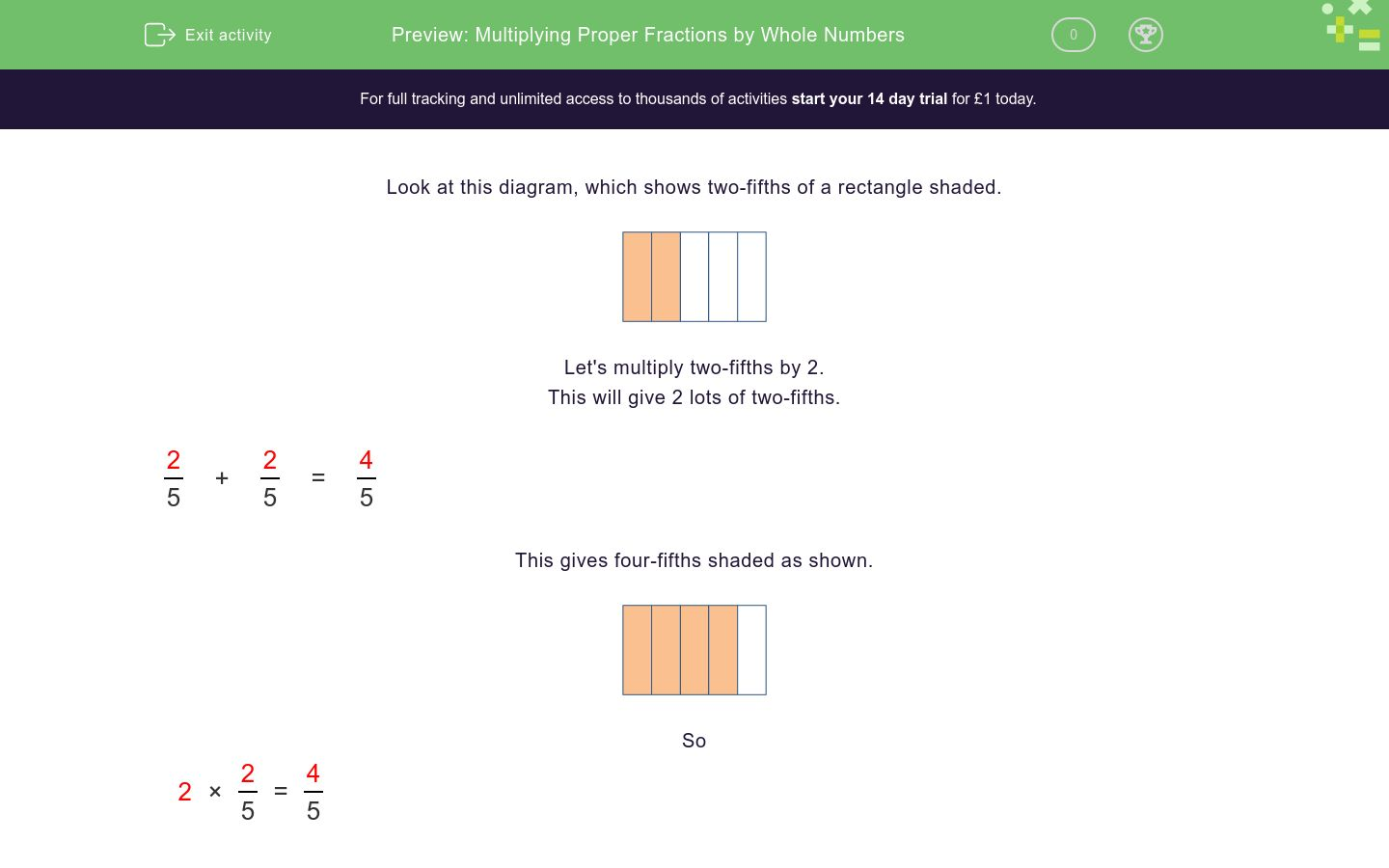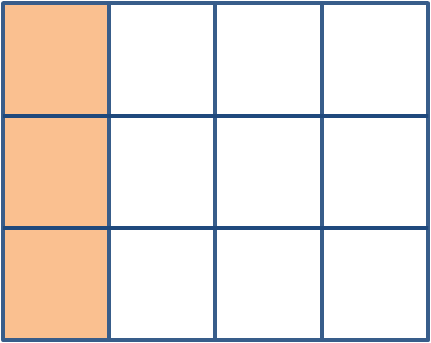# Multiplying Proper Fractions by Whole Numbers

In this worksheet, students use diagrams to multiply proper fractions by whole numbers. Answers may involve mixed numbers.Key stage:  KS 2

Curriculum topic:   Number: Fractions, Decimals and Percentages

Curriculum subtopic:   Multiply Fractions

Difficulty level:### QUESTION 1 of 10

Look at this diagram, which shows two-fifths of a rectangle shaded.Let's multiply two-fifths by 2.

This will give 2 lots of two-fifths.

 2 + 2 = 4 5 5 5

This gives four-fifths shaded as shown.So

 2 × 2 = 4 5 5

Now let's multiply two-fifths by 3.

This will be 3 lots of two-fifths.

 2 + 2 + 2 = 6 5 5 5 5

This gives six-fifths shaded as shown.So

 3 × 2 = 6 = 1 1 5 5 5Use the diagram to work out:

 2 × 1 = ? 4 4

(Just write the missing number shown by the ? mark.)Use the diagram to work out:

 2 × 2 = ? 6 6

(Just write the missing number shown by the ? mark.)Use the diagram to work out:

 2 × 2 = ? 8 8

(Just write the missing number shown by the ? mark.)Use the diagram to work out:

 3 × 2 = ? 8 8

(Just write the missing number shown by the ? mark.)Use the diagram to work out:

 3 × 3 = ? 12 12

(Just write the missing number shown by the ? mark.)Use the diagram to work out:

 5 × 1 = 5 = 1 ? 4 4 4

(Just write the missing number shown by the ? mark.)Use the diagram to work out:

 4 × 2 = 8 = 1 ? 6 6 6

(Just write the missing number shown by the ? mark.)Watch out, more numbers are missing. Use the diagram to work out:

 5 × 2 = = 1 ? 6 6 6

(Just write the missing number shown by the ? mark.)Use the diagram to work out:

 5 × 2 = = 1 ? 8 8 8

(Just write the missing number shown by the ? mark.)Use the diagram to work out:

 7 × 2 = = 1 ? 8 8 8

(Just write the missing number shown by the ? mark.)

• Question 1Use the diagram to work out:

 2 × 1 = ? 4 4

(Just write the missing number shown by the ? mark.)

2
EDDIE SAYS
1/4 + 1/4 = 2/4
• Question 2Use the diagram to work out:

 2 × 2 = ? 6 6

(Just write the missing number shown by the ? mark.)

4
EDDIE SAYS
2/6 + 2/6 = 4/6
• Question 3Use the diagram to work out:

 2 × 2 = ? 8 8

(Just write the missing number shown by the ? mark.)

4
EDDIE SAYS
2/8 + 2/8 = 4/8
• Question 4Use the diagram to work out:

 3 × 2 = ? 8 8

(Just write the missing number shown by the ? mark.)

6
EDDIE SAYS
2/8 + 2/8 + 2/8 = 6/8
• Question 5Use the diagram to work out:

 3 × 3 = ? 12 12

(Just write the missing number shown by the ? mark.)

9
EDDIE SAYS
3/12 + 3/12 + 3/12 = 9/12
• Question 6Use the diagram to work out:

 5 × 1 = 5 = 1 ? 4 4 4

(Just write the missing number shown by the ? mark.)

1
EDDIE SAYS
5/4 = 1 and a quarter
• Question 7Use the diagram to work out:

 4 × 2 = 8 = 1 ? 6 6 6

(Just write the missing number shown by the ? mark.)

2
EDDIE SAYS
8/6 = 1 and two sixths
• Question 8Watch out, more numbers are missing. Use the diagram to work out:

 5 × 2 = = 1 ? 6 6 6

(Just write the missing number shown by the ? mark.)

4
EDDIE SAYS
10/6 = 1 and four sixths
• Question 9Use the diagram to work out:

 5 × 2 = = 1 ? 8 8 8

(Just write the missing number shown by the ? mark.)

2
EDDIE SAYS
10/8 = 1 and two eighths
• Question 10Use the diagram to work out:

 7 × 2 = = 1 ? 8 8 8

(Just write the missing number shown by the ? mark.)

6
EDDIE SAYS
14/8 = 1 and six eighths
---- OR ----

Sign up for a £1 trial so you can track and measure your child's progress on this activity.

### What is EdPlace?

We're your National Curriculum aligned online education content provider helping each child succeed in English, maths and science from year 1 to GCSE. With an EdPlace account you’ll be able to track and measure progress, helping each child achieve their best. We build confidence and attainment by personalising each child’s learning at a level that suits them.

Get started